# Percentages Problems

#### Number of problems found: 670

• Car valueThe car loses value 15% every year. Determine a time (in years) when the price will be halved.
• Sum 10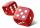What is the probability that two dice throw twice in a row will result the sum of 10?
• VacationTomas spent 60% of his savings for his weekly vacation. He was 32 € left. How many euros did he have before vacation?
• Ditch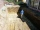The excavator dug 40% of the excavation in 2 hours 20 minutes. How long does it take him to dig the whole ditch?
• An investmentHow long will it take for an investment to double at a simple interest rate of 5.10% p. A. ? Express the answer in years and months, rounded up to the next month.
• ChocolateChocolate, which originally cost 1.5 euros, was increased by 40%. How many euros did it cost?
• Profit gainIf 5% more is gained by selling an article for Rs. 350 than by selling it for Rs. 340, the cost of the article is:
• Prices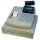The price of the product was increased by 35%. How many percents of the new price we have to make it cheaper so that its price is equal to the original price?A radioactive material loses 10% of its mass each year. What proportion will be left there after n=6 years?
• Rate or interestAt what rate percent will Rs.2000 amount to Rs.2315.25 in 3 years at compound interest?
• Efficiency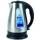What is the power output of kettle 2 kW with efficiency 90%?
• Salary increase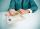Ms. Merry's salary increased by 15%, and that was 83 euros. What should pay before the increase?
• EnrollmentThe enrollment at a local college increased 4% over last year's enrollment of 8548. Find the increase in enrollment (x1) and the current enrollment (x2).
• PupilsThere are 350 girls in the school, and the other 30% of the total number of pupils are boys. How many pupils does the school have?
• Percent chaining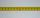Calculate 20% from 70% from 80 km
• Machine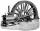Price of the new machine is € 62000. Every year is depreciated 15% of residual value. What will be the value of the machine after 3 years?
• DicesWe will throw two dice. What is the probability that the ratio between numbers on first and second dice will be 1:2?
• Deposit is pesosSally deposits Php.22,000 in her savings account. If the bank pays 1.5% interest per year, how much will she receive at the end of the year?
• Loan 4Mr santos borrowed Php.18,000 at 1.5% interest for one year. What was the total amount he paid for the end of the 1-year term?
• EmployeesThere are 1116 people working in three factory halls. In the first one, there are 18% more than the third, and 60 persons more than the second. How many employees work in individual halls?

Do you have an interesting mathematical word problem that you can't solve it? Submit a math problem, and we can try to solve it.

We will send a solution to your e-mail address. Solved examples are also published here. Please enter the e-mail correctly and check whether you don't have a full mailbox.

Please do not submit problems from current active competitions such as Mathematical Olympiad, correspondence seminars etc...